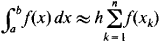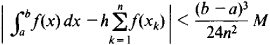# Rectangle Rule

## Rectangle Rule

a simple formula for the approximate evaluation of a definite integral. It has the formwhere h = (b -a)/n,xk = ξ + (k - 1)h, and aξa + h.

The most accurate form of the rectangle rule is the midpoint rule, in which ξ = a + h/2. If ǀf″ (x)ǀ < M on the interval [a, b], this formula satisfies the inequalityIn general, the other forms of the rectangle rule are less accurate. Therefore, in place of formulas in which ξ = a and ξ = a + h, it is preferable to use the arithmetic means of these formulas, since the error will then be at most (b — α)2M /1 2n2. This method is called the trapezoid rule. If both sides of the rectangle rules for ξ = a + h/2, ξ = a, and ξ = a + h are multiplied by 2/3, 1/6, and 1/6, respectively, and then added together, a more accurate formula for approximate integration is obtained. This formula is called Simpson’s rule. The error in this case is at most (b — a)5N/2,880n4, where N is the maximum value of ǀf(4)(x)ǀ on the interval [a, b].

Site: Follow: Share:
Open / Close